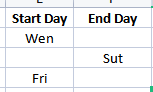# How to use AND & OR conditions in "if" Activity?

Hi All,

I would like to know how to give expression in “if condition”.

Condition is : if start day or end day is empty then select the day whichever is non-empty.

For example :So here , Robot should select Wen, Sut, Fri

Say your are using a for loop for each row in datatable with these columns

then inside for loop you will have if and condition would be(Assumption id either one will have data)

`IsNullOrEmpty(CurrentRow("Start Day")) AndAlso CurrentRow("Start Day").ToSTring.Trim.Equals(String.Empty)`

And - Will check for both conditions
AndAlso - Will check the second condition only if the first condition is satisfied

This checks for Start Day is empty or null or is blank

So in then Use
`CurrentRow("End Day").TOString`
and in else
`CurrentRow("Start Day").ToString`

Hope this helps

cheers

Hello @Vaishnav_Tej

You can use the Else If activity in UiPath.

Thanks